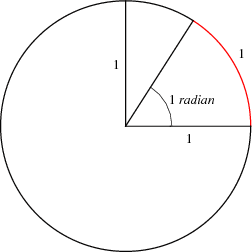ISRO | ISRO CS 2014 | Question 9

• Last Updated : 11 May, 2018

Let be the radius of the circle. What is the angle subtended by an arc of length at the center of the circle?
(A) 1 degree
(C) 90 degrees

Explanation: Radian describes the plane angle subtended by a circular arc as the length of the arc divided by the radius of the arc. One radian is the angle subtended at the center of a circle by an arc that is equal in length to the radius of the circle.Note that 1 radian = 57.2958 degree.

So, option (B) is correct.

Quiz of this Question

My Personal Notes arrow_drop_up